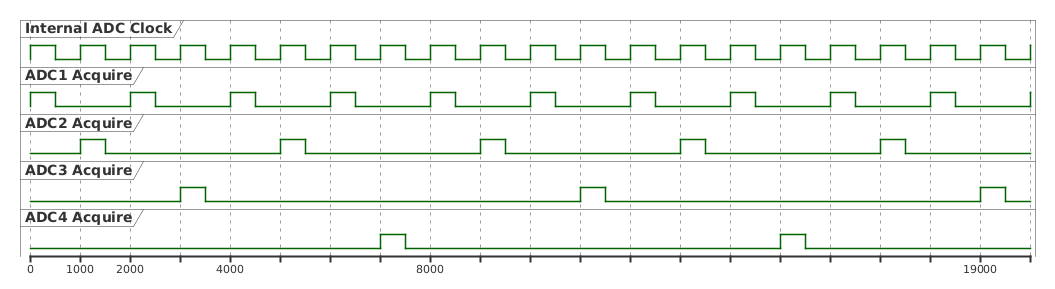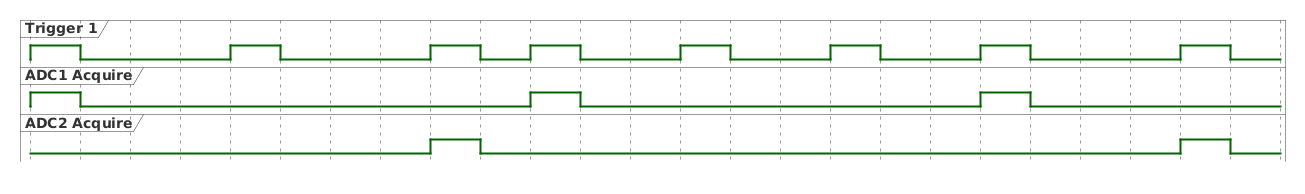By default, all ADC data acqusitions are triggered by an internal 1 MHz ADC clock. When the ADC is configured with the function:

void configureADC(uint8_t channel, uint16_t fire_every_us, uint16_t fire_delay, ... );

the fire_every_us sets how often the ADC should acquire a sample relative to this clock. If we configure two ADC channels with:

configureADC(1,3, 0, BIPOLAR_1250mV, getADC1); //±1.25V, runs every 3μsconfigureADC(2,6, 2, BIPOLAR_2500mV, getADC2); //±2.5V, runs every 6μs, with 2μ delay

then the following timing diagram shows when the ADC data acquisions are made.Some more complex timing situations can be setup this way, such as the following example that uses all 4 ADC channels and maximizes the 1 MSPS capability of the Quarto:

configureADC(1,2, 0, BIPOLAR_1250mV, getADC1); //±1.25V, runs every 2μsconfigureADC(2,4, 1, BIPOLAR_2500mV, getADC2); //±2.5V, runs every 4μs, with 1μ delayconfigureADC(2,8, 3, BIPOLAR_2500mV, getADC3); //±2.5V, runs every 8μs, with 3μ delayconfigureADC(2,8, 7, BIPOLAR_2500mV, getADC4); //±2.5V, runs every 8μs, with 7μ delay## Max Sample Rate​

The Quarto cannot acquire data faster than 1 MSPS, or once per µs. To make sure this limit is not broken, when setting the fire_every_us for each ADC, the following equation must be true:

$\sum_{i=1}^{4}\frac{1}{\text{fire\_every}\_us_{ADCi}} \le 1$

So, for example, if all four channels were configured to fire every 4µs, then the summation would be $\frac{1}{4} + \frac{1}{4} + \frac{1}{4}+ \frac{1}{4} =1$, indicating that we are using the maximum acquision speed of the Quarto. Likewise, the above example with fire_every_us values of 2,4,8,8 also maximizes the acqusition rate. Any channels not used can be ignored in the summation. So two active channels sampling every 2µs is a valid configuration.

In applications where the ADC acqisition should be sychronized with external events, we can input an ADC trigger signal into the Quarto and use that instead of the internal ADC clock.

Firmware Requirement

These functions require firmware version 1.32.52 or higher. You can check your firmware version with the Arduino example "Device Info" under the "Testing" category. If you have an earlier firmware revision, please contact qNimble to get upgrade instructions.

Use the function

bool useExtADCClock(bool active, uint8_t trigger_pin);

to activing using an external source (from trigger 1 or trigger 2) as the ADC clock. After running this function, the ADC timing diagrams will look the same as above, the only difference is that a) the clock will be externally provided and b) the clock does not need to be 'clock' but can be a series of triggers. For example, if we run:

useExtADCClock(true, 1);configureADC(1,3, 0, BIPOLAR_1250mV, getADC1); //±1.25V, runs every 3μsconfigureADC(2,6, 2, BIPOLAR_2500mV, getADC2); //±2.5V, runs every 6μs, with 2μ delay

we will get the following timingwhich is the same timing as above, but stretched out by the trigger 1 signal. If you want to use a single ADC channel and have it acquire data whenever the trigger signal fires, simply set the fire_every_us to 1.

note

Even if an external ADC clock is used and operating at less than 1 MHz, the fire_every_us values must be set according to the limits described above.